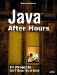The actionPerformed method handles menu selections, setting the appropriate drawing flags as needed. This method handles the File menu's Open item by using the ImageIO class to open an image file: ` public void actionPerformed(ActionEvent e) { if(e.getSource() == openMenuItem){ try{ dialog.setMode(FileDialog.LOAD); dialog.setVisible(true); if(dialog.getFile() != ""){ File inputFile = new File(dialog.getDirectory() + dialog.getFile()); bufferedImage = ImageIO.read(inputFile); if(bufferedImage != null){ image = createImage(bufferedImage.getWidth(), bufferedImage.getHeight()); Graphics2D g2d = (Graphics2D) image.getGraphics(); g2d.drawImage(bufferedImage, null, 0, 0); imageWidth = bufferedImage.getWidth(); imageHeight = bufferedImage.getHeight(); setSize(imageWidth + 100, imageHeight + 90); repaint(); } } }catch(Exception exp){ System.out.println(exp.getMessage()); } } . . . ` If the user selects the Save As menu item, Painter displays a File Save dialog box and saves the file in the same way that the Graphicizer application did: ` if(e.getSource() == saveMenuItem){ dialog.setMode(FileDialog.SAVE); dialog.setVisible(true); try{ if(dialog.getFile() != ""){ String outfile = dialog.getFile(); File outputFile = new File(dialog.getDirectory() + outfile); bufferedImage.createGraphics().drawImage(image, 0, 0, this); ImageIO.write(bufferedImage, outfile.substring(outfile.length() - 3, outfile.length()), outputFile); } } catch(Exception ex){ System.out.println(ex.getMessage()); } } . . . ` If the user selects the File menu's New item, the application creates new BufferedImage and Image objects as well as sets the recorded location at which the mouse button was pressed (the Point object start) and the location at which the mouse button was released (end) to negative values so no graphics shape will be drawn when the window is repainted: ` if(e.getSource() == newMenuItem){ bufferedImage = new BufferedImage (300, 300, BufferedImage.TYPE_INT_BGR ); image = createImage(300, 300); start.x = -20; start.y = -20; end.x = -20; end.y = -20; repaint(); } . . . ` When the user selects an item in the Draw or Effects menu, Painter sets the appropriate internal Boolean flags to match. Because those menu items are CheckboxMenuItem objects, they will call a method named itemStateChanged, which is where the code is that sets the various drawing and effects flags. If the user selects the Draw menu's Draw lines menu item, the code calls a method named setFlagsFalse to reset the drawing flags. It then sets the line Boolean flag to TRue, puts a check mark in front of the Draw lines menu item, and resets the start and end locations of the mouse to begin drawing with lines, like this: ` public void itemStateChanged(ItemEvent e) { if(e.getSource() == linesMenuItem){ setFlagsFalse(); line = true; linesMenuItem.setState(true); start.x = -20; start.y = -20; end.x = -20; end.y = -20; } . . . ` Here's the setFlagsFalse method: ` void setFlagsFalse() { rounded = false; line = false; ellipse = false; rectangle = false; draw = false; text = false; linesMenuItem.setState(false); ellipsesMenuItem.setState(false); rectanglesMenuItem.setState(false); roundedMenuItem.setState(false); freehandMenuItem.setState(false); textMenuItem.setState(false); } ` And here's how the other drawing flagsellipse, rectangle, rounded (for rounded rectangles) and draw (for freehand drawing)are set: ` if(e.getSource() == ellipsesMenuItem){ setFlagsFalse(); ellipse = true; ellipsesMenuItem.setState(true); start.x = -20; start.y = -20; end.x = -20; end.y = -20; } if(e.getSource() == rectanglesMenuItem){ setFlagsFalse(); rectangle = true; rectanglesMenuItem.setState(true); start.x = -20; start.y = -20; end.x = -20; end.y = -20; } if(e.getSource() == roundedMenuItem){ setFlagsFalse(); rounded = true; roundedMenuItem.setState(true); start.x = -20; start.y = -20; end.x = -20; end.y = -20; } if(e.getSource() == freehandMenuItem){ setFlagsFalse(); draw = true; freehandMenuItem.setState(true); start.x = -20; start.y = -20; end.x = -20; end.y = -20; } . . . ` Here's how the effects flags are set when the user selects an item from the Effects menu: ` if(e.getSource() == solidMenuItem){ solid = !solid; if(solid){ texture = false; shade = false; } solidMenuItem.setState(solid); gradientMenuItem.setState(shade); textureMenuItem.setState(texture); plainMenuItem.setState(false); start.x = -20; start.y = -20; end.x = -20; end.y = -20; } if(e.getSource() == gradientMenuItem){ shade = !shade; if(shade){ solid = false; texture = false; } solidMenuItem.setState(solid); gradientMenuItem.setState(shade); textureMenuItem.setState(texture); plainMenuItem.setState(false); start.x = -20; start.y = -20; end.x = -20; end.y = -20; } if(e.getSource() == textureMenuItem){ texture = !texture; if(texture){ shade = false; solid = false; } solidMenuItem.setState(solid); gradientMenuItem.setState(shade); textureMenuItem.setState(texture); plainMenuItem.setState(false); start.x = -20; start.y = -20; end.x = -20; end.y = -20; } if(e.getSource() == transparentMenuItem){ transparent = !transparent; transparentMenuItem.setState(transparent); start.x = -20; start.y = -20; end.x = -20; end.y = -20; } if(e.getSource() == textMenuItem){ textDialog.setVisible(true); drawText = textDialog.data; setFlagsFalse(); text = true; textMenuItem.setState(true); start.x = -20; start.y = -20; end.x = -20; end.y = -20; } if(e.getSource() == thickMenuItem){ thick = thickMenuItem.getState(); start.x = -20; start.y = -20; end.x = -20; end.y = -20; } if(e.getSource() == plainMenuItem){ solidMenuItem.setState(false); gradientMenuItem.setState(false); textureMenuItem.setState(false); transparentMenuItem.setState(false); plainMenuItem.setState(true); shade = false; solid = false; transparent = false; texture = false; start.x = -20; start.y = -20; end.x = -20; end.y = -20; } if(e.getSource() == shadowMenuItem){ shadow = shadowMenuItem.getState(); start.x = -20; start.y = -20; end.x = -20; end.y = -20; } } ` That's all you really have to do in the menu itemsjust set the Boolean flags that the rest of the program will check. After the user selects his drawing and effects options, he can use the mouse to draw figures.Java After Hours: 10 Projects Youll Never Do at Work
ISBN: 0672327473
EAN: 2147483647
Year: 2006
Pages: 128

Similar book on Amazon